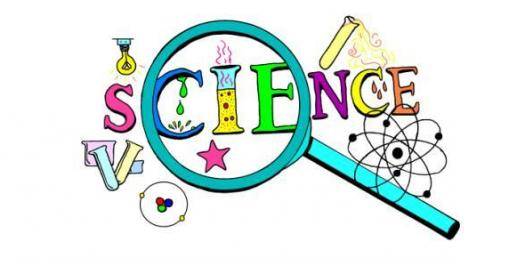# Science Sol 4.8 Test

21 Questions | Total Attempts: 355SettingsChoose the best answer for each question. Write the letter of your answer on the sheet provided. Do not write on the test.

• 1.
The rotation of the Earth on its axis causes --
• A.

Seasons

• B.

Years

• C.

Months

• D.

Days

• 2.
Earth’s _____ is involved in determining the length of a year.
• A.

Revolution

• B.

Rotation

• C.

Axis

• D.

Temperature

• 3.
The moon revolves around--
• A.

Itself

• B.

The Earth

• C.

The sun

• D.

The solar system

• 4.
Which of these statements is NOT true?
• A.

Earth spins on its axis.

• B.

Earth revolves around the sun.

• C.

Earth is a planet.

• D.

Earth rotates around the moon

• 5.
Earth revolves around the sun in a/an:
• A.

Eclipse

• B.

Axis.

• C.

Ellipse.

• D.

Circle.

• 6.
As Earth revolves around the sun, what causes the seasons?
• A.

The shape of Earth’s orbit

• B.

The tilt of Earth’s axis

• C.

The position of the moon

• D.

The temperature of the sun’s heat

• 7.
If it is hot year-round where you live, then where do you live?
• A.

In the Northern Hemisphere

• B.

In the Southern Hemisphere

• C.

Near the equator

• D.

Near the North Pole

• 8.
The apparent changes of the moon’s shape during the month are called:
• A.

Cycles.

• B.

Phases.

• C.

Revolutions.

• D.

Days.

• 9.
When the moon is seen from the Earth as a whole circle, it is called a—
• A.

Full moon

• B.

Crescent moon

• C.

New moon

• D.

Half moon

• 10.
Which of the following statements is NOT true?
• A.

The moon is smaller than Earth.

• B.

The moon revolves around Earth in about 4 weeks.

• C.

Earth revolves around the sun in about 52 weeks.

• D.

The moon is heavier than Earth.

• 11.
The Earth is very different from other objects in space, because it has the most—
• A.

Solid rock

• B.

Volcanoes

• C.

Liquid water

• D.

High winds

• 12.
The sun is a—
• A.

Planet

• B.

Moon

• C.

Asteroid

• D.

Star

• 13.
Approximately how old is the sun?
• A.

Thousand years

• B.

Hundreds years

• C.

Millions years

• D.

Billion years

• 14.
Which of the following statements is NOT true?
• A.

The Earth is larger than the moon

• B.

The moon is smaller than the sun

• C.

The sun is the largest body in our solar system.

• D.

The Earth is larger than the sun.

• 15.
Which of the following is small and rocky with extreme temperatures and no water?
• A.

The moon

• B.

The Earth

• C.

Sun

• D.

Stars

• 16.
Which of the following is a yellow ball of burning gases that provides heat and light for the nine planets that orbit it?
• A.

Earth

• B.

Moon

• C.

Comets

• D.

Sun

• 17.
On which of the following does life exist?
• A.

Meteors

• B.

The Earth

• C.

The moon

• D.

Sun

• 18.
What group of people have helped us most to understand the moon, the sun, and earth systems?
• A.

Farmers and fishermen

• B.

Airline pilots

• C.

Engineers

• D.

Astronomers and astronauts

• 19.
The NASA Apollo missions helped us to understand—
• A.

The sun

• B.

The moon

• C.

The Earth

• D.

Mars

• 20.
Many years ago, scientists such as Aristotle and Ptolemy thought that ________ was at the center of the solar system.
• A.

The moon

• B.

The Earth

• C.

The sun

• D.

A meteorite

• 21.
Scientists such as Copernicus and Galileo helped to prove that the ________ is in the center of the solar system.
• A.

Sun

• B.

Moon

• C.

Earth

• D.

Comet

Related TopicsBack to top